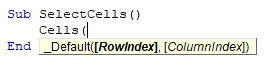# VBA Cells Function – Cells, Worksheet.Cells, & Range.Cells

The VBA Cells Function allows you to do two things:

1. Reference all cells in a worksheet
2. Reference a specific cell

This is similar to the VBA Range Object.

## Syntax of VBA Cells

The traditional syntax of the VBA Cells function is:This type of syntax is called R1C1 syntax as opposed to the A1 syntax of a column letter and then a row number which is used when using the Range Object in Excel VBA.

Therefore, to select cell C3 we can type the following code:

``Cells(3, 4).Select``

this will move the Excel pointer to cell C4 in your worksheet.

If we wish to use the cells function to populate a specific cell with some text, and then format the cell, we can write some code like this example below:

``````Sub PopulateCell()
Cells(2, 2) = "Sales Analysis for 2022"
Cells(2, 2).Font.Bold = True
Cells(2, 2).Font.Name = "Arial"
Cells(2, 2).Font.Size = 12
End Sub``````

This will populate cell B2 with text and then format the cell.

## Worksheet.Cells

If we wish to select the entire worksheet using the Cells Function, we can type the following:

``Worksheets("Sheet1").Cells.Select``

This will select all the cells in Sheet 1 in the same way that pressing CTRL + A on the keyboard would do so.

## Range.Cells

We can also use the cells function to refer to a specific cell within a range of cells.

``Range("A5:B10").Cells(2, 2).Select``

This will actually refer to the cell that has the address of  B6 in the original range (A5:B10) as B6 is in the second row and second column of that range.

## Looping through a Range with the Cells Function

Now that we understand the Cells Function, we can use it to perform some tasks in VBA Loops.

For example, if we want to format a range of cells depending on their value, we can loop through the range using the cells function.

``````Sub FormatCells()
Dim x As Integer
For x = 1 To 10
If Cells(x, 1) > 5 Then
Cells(x, 1).Interior.Color = vbRed
End If
Next x
End Sub``````

This code will start at cell A1 and loop through to cell A10, changing the background color to red if the value in the cell is greater than 5.

If we wish to take this to another level, to loop through columns as well as rows, we can add in a further loop to the code to increase the columns that the loops looks at.

``````Sub FormatCells()
Dim x As Integer
Dim y As Integer
For x = 1 To 10
For y = 1 To 5
If Cells(x, y) > 5 Then
Cells(x, y).Interior.Color = vbRed
End If
Next y
Next x
End Sub``````

Now the loop will start at cell A5 but will continue all the way through to cell E10.

We can also use the Cells function to loop through a specific range of cells

``````Sub LoopThrouRange()
Dim rng As Range
Dim x As Integer
Dim y As Integer
Set rng = Range("B2:D8")
For x = 1 To 7
For y = 1 To 3
If rng.Cells(x, y) > 5 Then
rng.Cells(x, y).Interior.Color = vbRed
End If
Next y
Next x
End Sub``````

This loop would only look inside the range B2:D8, and then loop through the rows and columns of that particular range. Remember that when the range is supplied to the Cells Function, the Cells Function will only look within that range and not at the entire worksheet.

Stop searching for VBA code online. Learn more about AutoMacro - A VBA Code Builder that allows beginners to code procedures from scratch with minimal coding knowledge and with many time-saving features for all users!